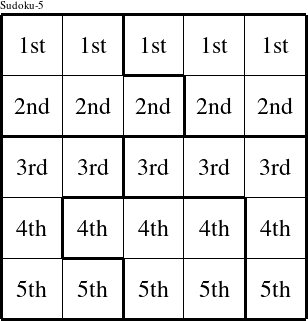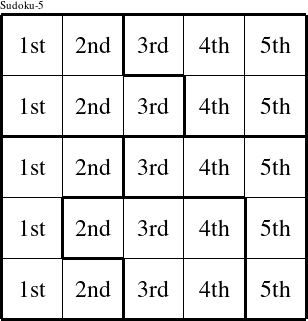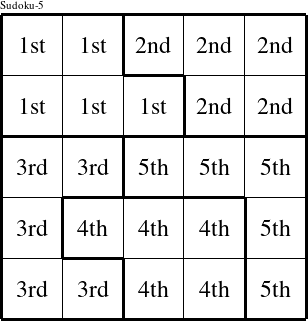#### Printable version

Detailed instructions for working a group puzzle of type 'Brian'

This is a signature puzzle named 'Brian'

The 5 elements in each group of this type of puzzle are: B, R, I, A, and N.

Every group in the puzzle must contain exactly this set of elements.

####In a Brian puzzle, each row is a group numbered as shown in the figure above.

####In a Brian puzzle, each column is a group numbered as shown in the figure above.

####In a Brian puzzle, each pentomino is a group numbered as shown in the figure above.